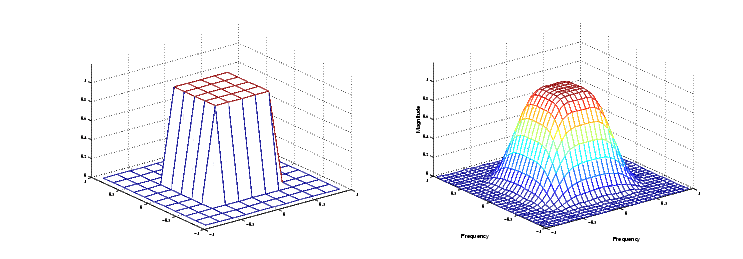Image Processing Toolbox User's GuideWindowing Method

The windowing method involves multiplying the ideal impulse response with a window function to generate a corresponding filter, which tapers the ideal impulse response. Like the frequency sampling method, the windowing method produces a filter whose frequency response approximates a desired frequency response. The windowing method, however, tends to produce better results than the frequency sampling method.

The toolbox provides two functions for window-based filter design, `fwind1` and `fwind2`. `fwind1` designs a two-dimensional filter by using a two-dimensional window that it creates from one or two one-dimensional windows that you specify. `fwind2` designs a two-dimensional filter by using a specified two-dimensional window directly.

`fwind1` supports two different methods for making the two-dimensional windows it uses:

• Transforming a single one-dimensional window to create a two-dimensional window that is nearly circularly symmetric, by using a process similar to rotation
• Creating a rectangular, separable window from two one-dimensional windows, by computing their outer product

The example below uses `fwind1` to create an 11-by-11 filter from the desired frequency response `Hd`. Here, the `hamming` function from the Signal Processing Toolbox is used to create a one-dimensional window, which `fwind1` then extends to a two-dimensional window.

• ```Hd = zeros(11,11); Hd(4:8,4:8) = 1;
[f1,f2] = freqspace(11,'meshgrid');
mesh(f1,f2,Hd), axis([-1 1 -1 1 0 1.2]), colormap(jet(64))
h = fwind1(Hd,hamming(11));
figure, freqz2(h,[32 32]), axis([-1 1 -1 1 0 1.2])```

Desired Two-Dimensional Frequency Response (left) and Actual Two-Dimensional Frequency Response (right)Frequency Sampling Method Creating the Desired Frequency Response Matrix